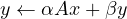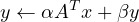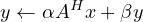#### 4.12 psb_spmm — Sparse Matrix by Dense Matrix Product

This subroutine computes the Sparse Matrix by Dense Matrix Product:(1)(2)(3)

where:

x
is the global dense matrix x:,:
y
is the global dense matrix y:,:
A
is the global sparse matrix A

 A, x, y, α, β Subroutine Short Precision Real psb_spmm Long Precision Real psb_spmm Short Precision Complex psb_spmm Long Precision Complex psb_spmm

Table 12: Data types

call psb_spmm(alpha, a, x, beta, y, desc_a, info)
call psb_spmm(alpha, a, x, beta, y,desc_a, info, trans, work)

Type:
Synchronous.
On Entry
alpha
the scalar α.
Scope: global
Type: required
Intent: in.
Specified as: a number of the data type indicated in Table 12.
a
the local portion of the sparse matrix A.
Scope: local
Type: required
Intent: in.
Specified as: an object of type psb_Tspmat_type.
x
the local portion of global dense matrix x.
Scope: local
Type: required
Intent: in.
Specified as: a rank one or two array or an object of type psb_T_vect_type containing numbers of type specified in Table 12. The rank of x must be the same of y.
beta
the scalar β.
Scope: global
Type: required
Intent: in.
Specified as: a number of the data type indicated in Table 12.
y
the local portion of global dense matrix y.
Scope: local
Type: required
Intent: inout.
Specified as: a rank one or two array or an object of type psb_T_vect_type containing numbers of type specified in Table 12. The rank of y must be the same of x.
desc_a
contains data structures for communications.
Scope: local
Type: required
Intent: in.
Specified as: an object of type psb_desc_type.
trans
indicates what kind of operation to perform.
trans = N
the operation is specified by equation 1
trans = T
the operation is specified by equation 2
trans = C
the operation is specified by equation 3

Scope: global
Type: optional
Intent: in.
Default: trans = N
Specified as: a character variable.

work
work array.
Scope: local
Type: optional
Intent: inout.
Specified as: a rank one array of the same type of x and y with the TARGET attribute.
On Return
y
the local portion of result matrix y.
Scope: local
Type: required
Intent: inout.
Specified as: an array of rank one or two containing numbers of type specified in Table 12.
info
Error code.
Scope: local
Type: required
Intent: out.
An integer value; 0 means no error has been detected.